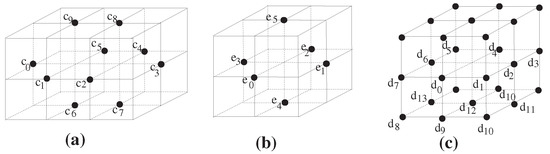Previous Article in Journal
Constructing Some Logical Algebras with Hoops
Previous Article in Special Issue
Approximations of Fixed Points in the Hadamard Metric Space CATp(0)
Open AccessArticle

# Fixed Point Theory for Digital k-Surfaces and Some Remarks on the Euler Characteristics of Digital Closed Surfaces

bySang-Eon Han
Department of Mathematics Education, Institute of Pure and Applied Mathematics, Jeonbuk National University, Jeonju-City Jeonbuk 54896, Korea
Mathematics 2019, 7(12), 1244; https://doi.org/10.3390/math7121244
Received: 12 November 2019 / Revised: 4 December 2019 / Accepted: 8 December 2019 / Published: 16 December 2019
(This article belongs to the Special Issue Fixed Point Theory and Related Nonlinear Problems with Applications)
The present paper studies the fixed point property (FPP) for closed k-surfaces. We also intensively study Euler characteristics of a closed k-surface and a connected sum of closed k-surfaces. Furthermore, we explore some relationships between the FPP and Euler characteristics of closed k-surfaces. After explaining how to define the Euler characteristic of a closed k-surface more precisely, we confirm a certain consistency of the Euler characteristic of a closed k-surface and a continuous analog of it. In proceeding with this work, for a simple closed k-surface in $Z 3$ , say $S k$ , we can see that both the minimal 26-adjacency neighborhood of a point $x ∈ S k$ , denoted by $M k ( x )$ , and the geometric realization of it in $R 3$ , denoted by $D k ( x )$ , play important roles in both digital surface theory and fixed point theory. Moreover, we prove that the simple closed 18-surfaces $M S S 18$ and $M S S 18 ′$ do not have the almost fixed point property (AFPP). Consequently, we conclude that the triviality or the non-triviality of the Euler characteristics of simple closed k-surfaces have no relationships with the FPP in digital topology. Using this fact, we correct many errors in many papers written by L. Boxer et al. View Full-Text
Show FiguresFigure 1

MDPI and ACS Style

Han, S.-E. Fixed Point Theory for Digital k-Surfaces and Some Remarks on the Euler Characteristics of Digital Closed Surfaces. Mathematics 2019, 7, 1244.